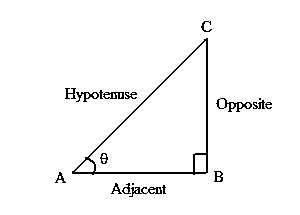# Sine Cosine Tangent Formula

To calculate the angle of a right triangle, sine cosine tangent formula is used. The ratio of the different sides of the triangle gives the sine, cosine, and tangent angles. Here, the hypotenuse is the longest side, the side opposite to the hypotenuse is the opposite side and the where both the sides rest is the adjacent side. A right angle looks like this:## Formulas for Sine, Cos, Tan

The Sine Cosine Tangent Formula is,

Angle Name Angle Formula
Sine Angle Formula Sin θ = Opposite side ⁄Hypotenuse
Cos Angle Formula Cos θ = Adjacent side⁄Hypotenuse
Tangent Angle Formula Tan θ = Opposite Side ⁄Adjacent Side

## Solved Examples

Question 1: Calculate the angle in a right triangle whose adjacent side and hypotenuse are 12 cm and 20 cm respectively? Solution:

Given,

Hypotenuse = 20 cm

cos θ = 12⁄20

θ = cos−1(0.6)

θ = 53.13

Question 2: If sin A = 21/29 and cos A = 20/ 29, then find the value of tan A.

Solution:
Given,
sin A = 21/29
cos A = 20/29
We know that,
sin θ = Opposite/Hypotenuse
Thus,
Opposite = 21
Hypotenuse = 29
= 21/20

Question 3: If sin A = ⅗, then find the value of cos A and tan A.

Solution:
Given,
sin A = Opposite/Adjacent = ⅗
By Pythagoras theorem,
(Hypotenuse)2 = (Opposite side)2 + (Adjacent side)2
52 = 32 + (Adjacent side)2
(Adjacent side)2 = 25 – 9 = 16
Adjacent side = √16 = 4
Therefore,
cos A = Adjacent/Hypotenuse = ⅘
tan A = Opposite/Adjacent = ¾

More topics in Sine Cosine Tangent Formulas
Sine Formula Cosine Formula
Tangent Formula Arctan Formula
Half Angle formula Double Angle Formulas